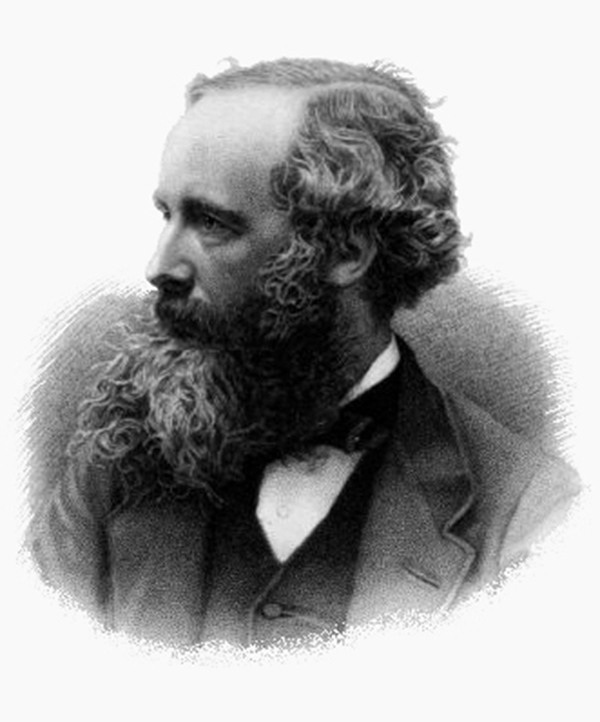# 8.1 Maxwell’s equations: electromagnetic waves predicted and

 Page 1 / 10
• Restate Maxwell’s equations.

The Scotsman James Clerk Maxwell (1831–1879) is regarded as the greatest theoretical physicist of the 19th century. (See [link] .) Although he died young, Maxwell not only formulated a complete electromagnetic theory, represented by Maxwell’s equations    , he also developed the kinetic theory of gases and made significant contributions to the understanding of color vision and the nature of Saturn’s rings.James Clerk Maxwell, a 19th-century physicist, developed a theory that explained the relationship between electricity and magnetism and correctly predicted that visible light is caused by electromagnetic waves. (credit: G. J. Stodart)

Maxwell brought together all the work that had been done by brilliant physicists such as Oersted, Coulomb, Gauss, and Faraday, and added his own insights to develop the overarching theory of electromagnetism. Maxwell’s equations are paraphrased here in words because their mathematical statement is beyond the level of this text. However, the equations illustrate how apparently simple mathematical statements can elegantly unite and express a multitude of concepts—why mathematics is the language of science.

## Maxwell’s equations

1. Electric field lines originate on positive charges and terminate on negative charges. The electric field is defined as the force per unit charge on a test charge, and the strength of the force is related to the electric constant ${\epsilon }_{0}$ , also known as the permittivity of free space. From Maxwell’s first equation we obtain a special form of Coulomb’s law known as Gauss’s law for electricity.
2. Magnetic field lines are continuous, having no beginning or end. No magnetic monopoles are known to exist. The strength of the magnetic force is related to the magnetic constant ${\mu }_{0}$ , also known as the permeability of free space. This second of Maxwell’s equations is known as Gauss’s law for magnetism.
3. A changing magnetic field induces an electromotive force (emf) and, hence, an electric field. The direction of the emf opposes the change. This third of Maxwell’s equations is Faraday’s law of induction, and includes Lenz’s law.
4. Magnetic fields are generated by moving charges or by changing electric fields. This fourth of Maxwell’s equations encompasses Ampere’s law and adds another source of magnetism—changing electric fields.

Maxwell’s equations encompass the major laws of electricity and magnetism. What is not so apparent is the symmetry that Maxwell introduced in his mathematical framework. Especially important is his addition of the hypothesis that changing electric fields create magnetic fields. This is exactly analogous (and symmetric) to Faraday’s law of induction and had been suspected for some time, but fits beautifully into Maxwell’s equations.

Symmetry is apparent in nature in a wide range of situations. In contemporary research, symmetry plays a major part in the search for sub-atomic particles using massive multinational particle accelerators such as the new Large Hadron Collider at CERN.

## Making connections: unification of forces

Maxwell’s complete and symmetric theory showed that electric and magnetic forces are not separate, but different manifestations of the same thing—the electromagnetic force. This classical unification of forces is one motivation for current attempts to unify the four basic forces in nature—the gravitational, electrical, strong, and weak nuclear forces.

Got questions? Join the online conversation and get instant answers!ByBy Qqq Qqq